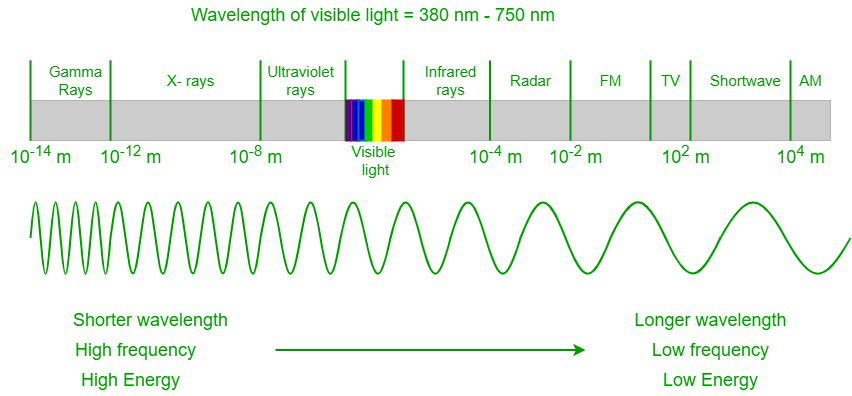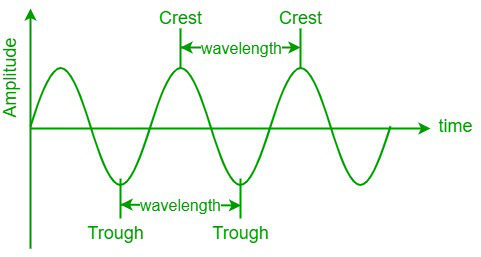# How to calculate the Wavelength of the Light?

• Last Updated : 15 May, 2022

All electromagnetic radiation is light, and it occurs over an extremely wide range of wavelengths, from high-energy gamma waves with shorter wavelengths to low-energy radio waves with longer wavelengths. But the human eye can detect only a small portion of the radiation, and that portion is referred to as visible light. In an electromagnetic spectrum, the visible spectrum lies in between the infrared spectrum and the UV spectrum. Visible light ranges between a wavelength of 400 nm and 700 nm. The human eye cannot detect other electromagnetic radiation as the radiation has either large or small wavelengths and is out of biological limitations.Electromagnetic spectrum

When a visible spectrum travels through a prism, the light gets separated into a spectrum of colors of different wavelengths. The violet color has the shortest wavelength of around 380 nm, and the red color has the longest wavelength of around 700 nm. Our eyes can detect the outer-most layer of the sun, the corona, in visible light.

### Wavelength

A wavelength is one of the properties of a wave and is defined as the distance between the two successive crests or troughs of a wave, where a crest is the highest point of the wave, and a trough is the lowest point of the wave. Since wavelength is a distance or length between two points, it is measured in meters, centimeters, millimeters, micrometers, etc. It is denoted by the symbol Lambda ‘λ’.Wave

Frequency

Frequency (f) is defined as the total number of wave cycles or oscillations produced per unit of time. Frequency is measured in terms of Hertz (Hz) or s-1.

The formula for the frequency:

Frequency (f) = 1/period(T)

f = 1/T

• A period is defined as the time taken to complete an oscillation.
• From the equation of frequency, we can conclude that the frequency of a wave is inversely proportional to its period.
• 1 Hertz = 1 oscillation/second

Wave velocity

The velocity of a wave or wave velocity is defined as the distance traveled by the wave in a unit of time. The S.I. unit of wave velocity is ms-1.

• Light travels with a speed in the vacuum of 29,97,92,458 m/s, i.e., approximately 3 × 108 m/s, and it is represented by the symbol c.

### Wavelength of the light

We know that light possesses the characteristics of both a wave and a particle. So, the wavelength of a light wave is given as;

λ =Where λ is the wavelength of light

c is the velocity of light and

f is the frequency of the light

The energy of a photon is given as,

E = h × f =Where E is the energy of a photon

h is the Planck’s constant i.e., h = 6.64 × 10-34 joule-second

Wavelength, Frequency, and Energy of the visible light spectrum

### Sample Problems

Problem 1: Calculate the wavelength of the visible light with a frequency of 5.36 × 1014 Hz.

Solution:

Given the frequency of light = 5.36 × 1014 Hz

We know, that the velocity of light (c) = 3 × 108 m/s

Now, the wavelength of light (λ) =⇒ λ =⇒ λ = 5.60 × 10-7 m

Hence, the wavelength is 5.60 × 10-7 m

Problem 2: If a microwave oven emits microwave energy of 1.64 × 10-24 J, then calculate the wavelength of the microwave emitted.

Solution:

Given data,

The energy of microwave emitted = 1.64 × 10-24 J

We know, that the energy of a photon =h = 6.64 × 10-34 joule-second

⇒ 1.64 × 10-24⇒ λ =⇒ λ = 12.146 × 10-2 m = 12.15 cm

Hence, the wavelength of the microwave emitted is 12.15 cm.

Problem 3: If a radio station broadcasts at a frequency of 555 kHz, then calculate the wavelength of radio waves emitted.

Solution:

Given,

Frequency of radio waves = 555 KHz

We know, that the velocity of light (c) = 3 × 108 m/s

Now, the wavelength of light (λ) =⇒ λ =⇒ λ = 540 m

Hence the wavelength of radio waves emitted is 540 m.

Problem 4: Calculate the wavelength of yellow light emitted from a sodium lamp at a frequency of 5.15 × 1014 Hz.

Solution:

Given,

The frequency of yellow light = 5.15 × 1014 Hz

We know, that the velocity of light (c) = 3 × 108 m/s

Now, the wavelength of light (λ) =⇒ λ =⇒ λ = 582.5 × 10-9 m = 582.5 nm

Hence, the wavelength of the yellow light is 582.5 nm.

Problem 5: Calculate the wavelength of a photon with an energy of 3.35 × 10-19 Joules.

Solution:

Given,

The energy of a photon = 3.35 × 10-19 Joules.

We know, that the energy of a photon =h = 6.64 × 10-34 joule-second

⇒ 3.35 × 10-19  =⇒ λ =⇒ λ= 5.94 × 10-7 m = 594 nm

Hence, the wavelength of the photon is 594 nm.

Problem 6: The broadcasting frequency of a radio station is 101 MHz. What will be the wavelength of the wave if the broadcast wave is an electromagnetic wave?

Solution:

Given data, Frequency of the wave = 101 MHz = 101 × 106 Hz

Speed of light = 3 × 108 m/s

Now, the wavelength of light (λ) = c/f

⇒ λ = (3 × 108)/(101 × 106)

⇒ λ = 2.97 m

Hence, the wavelength of the broadcast wave is 2.97m

My Personal Notes arrow_drop_up